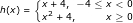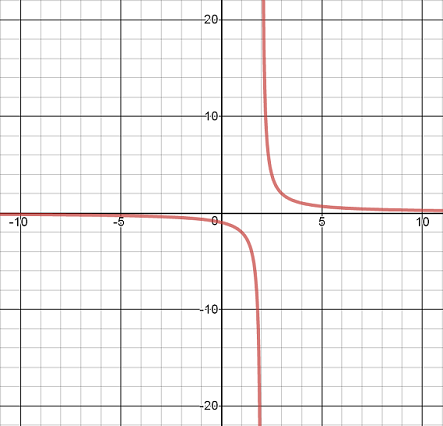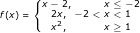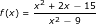# Function Continuity Flashcards

Function Continuity Flashcards
1/12 (missed) 0 0
Create Your Account To Continue Studying

As a member, you'll also get unlimited access to over 75,000 lessons in math, English, science, history, and more. Plus, get practice tests, quizzes, and personalized coaching to help you succeed.

Try it risk-free for 30 days. Cancel anytime.
###### Already registered? Login here for access
State the type of discontinuity.The function h(x) has a jump discontinuity at x = 0.
Got it
State the type of discontinuity shown below:The graph has an asymptotic discontinuity at x = 2.
Got it
Intermediate Value Theorem
If a function f(x) is continuous on the interval from a to b, then the function must take on every value between f(a) and f(b).
Got it
State x-values where discontinuity occurs.The function f(x) is discontinuous at x = 1.
Got it
Name the three types of discontinuities.
Point discontinuity, jump discontinuity, and asymptotic discontinuity.
Got it
Continuous Function
A continuous function can be traced without lifting the pencil. It has no undefined points or separated sections like steps or asymptotes.
Got it

### Ready to move on?

or choose a specific lesson: See all lessons in this chapter
12 cards in set

## Flashcard Content Overview

What makes a function continuous? And why do we need to know that it is continuous? This set of flashcards will help you review the properties of continuous functions and the Intermediate Value Theorem. You can also practice determining the various types of discontinuities that may occur in functions.

Front
Back
Continuous Function
A continuous function can be traced without lifting the pencil. It has no undefined points or separated sections like steps or asymptotes.
Name the three types of discontinuities.
Point discontinuity, jump discontinuity, and asymptotic discontinuity.
State x-values where discontinuity occurs.The function f(x) is discontinuous at x = 1.
Intermediate Value Theorem
If a function f(x) is continuous on the interval from a to b, then the function must take on every value between f(a) and f(b).
State the type of discontinuity shown below:The graph has an asymptotic discontinuity at x = 2.
State the type of discontinuity.The function h(x) has a jump discontinuity at x = 0.
Give the location of all discontinuities of the function f(x) = 5/(x2 - 4).
The function f(x) = 5/(x2 - 4) is discontinuous at vertical asymptotes located at x = -2 and x = 2.
You are told the following about the function g(x): g(-1) = 5, g(0) = 2, and g(1) = -3. State the interval on which the function must have a root according to the Intermediate Value Theorem.
The function g(x) must have a root on the interval from 0 and 1.
State what condition you must know or assume about every function to which you want to apply the Intermediate Value Theorem.
In order to apply the Intermediate Value Theorem, you must know or assume that the function with which you are working is continuous.
If f(x) = cos(3x), consider the function values at x = pi/9, x = 2pi/9, and x = pi/3 (measured in radians.) Using those values, state the interval on which f(x) must cross the x-axis.
The function f(x) = cos(3x) must cross the x-axis on the interval from pi/9 to 2pi/9.
On the interval from -10 to 10, the function f(x) has the following values: f(-10) = -7 and f(10) = 5. State the range of values that the function must take on in that interval.
The function must take on all of the values between -7 and 5 on the interval from -10 to 10.
State all discontinuities of the function:The function has a point discontinuity at x = 3 and an asymptotic discontinuity at x = -3.

To unlock this flashcard set you must be a Study.com Member.